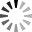# Position sizing techniques use for Risk Management

Position sizing techniques use for Risk Management💥💥Position sizing is a crucial aspect of quantitative trading. It refers to the process of determining the appropriate amount of capital to allocate to a particular trade or investment based on a set of predefined rules or strategies. Proper position sizing helps to manage risk and optimize returns.

💥Position sizing is an important aspect of quantitative trading that involves determining the appropriate amount of capital to allocate to a trade. There are several techniques that can be used to determine position size, including:

👉 1. Fixed Fractional Position Sizing: This is a popular position sizing technique that involves allocating a fixed percentage of the trading account balance to each trade. For example, if the fixed percentage is set at 2%, and the trading account has a balance of \$10,000, then the position size for each trade would be \$200. This technique helps to limit the risk exposure of the trading account to a small percentage of the account balance.

👉 2. Fixed Dollar Position Sizing: This technique involves allocating a fixed dollar amount to each trade. For example, if the fixed dollar amount is set at \$1,000, then the position size for each trade would be \$1,000. This technique is suitable for traders who have a fixed amount of capital to trade with and want to limit their risk exposure.

👉 3. Volatility-based Position Sizing: This technique involves adjusting the position size based on the volatility of the underlying asset. The position size is increased for assets with lower volatility and decreased for assets with higher volatility. This helps to ensure that the risk exposure is proportional to the volatility of the asset.

👉 4. Optimal f Position Sizing: This technique involves calculating the optimal fraction of the trading account to allocate to each trade based on the expected return and risk of the trade. The optimal fraction is calculated using a mathematical formula that takes into account the probability of the trade being successful and the potential loss if the trade is unsuccessful.

👉 5. Kelly Criterion Position Sizing: This technique involves using the Kelly criterion formula to calculate the optimal position size for each trade. The Kelly criterion takes into account the probability of success, the potential return, and the potential loss of each trade to determine the optimal position size.

👉 6. Percentage of portfolio: This technique involves allocating a percentage of the portfolio to each trade, based on the portfolio's value. For example, an investor may allocate 5% of their portfolio to each trade, regardless of the asset's price.

👉 7. Risk-based position sizing: This technique involves allocating a position size based on the amount of risk an investor is willing to take on. The position size is determined by the maximum amount of risk an investor is willing to take on per trade. For example, an investor may be willing to risk 1% of their portfolio on each trade, which would determine the position size.

👉 8. Monte Carlo simulation: This technique involves using a simulation to determine the optimal position size based on various scenarios and outcomes. This approach can help to account for uncertainty and risk in the trading strategy.

💥💥Proper position sizing is essential for effective risk management and maximizing returns in quantitative trading. Traders should carefully consider their trading strategies and risk tolerance when choosing a position sizing technique. It is also important to monitor and adjust position sizes regularly to account for changes in market conditions and risk exposure.

Tags:

 Thanks:

Attach files by dragging & dropping, , or pasting from the clipboard.